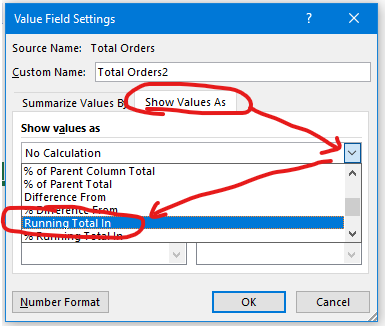Search

# Running TotalsCalculating the running total for a set of data is one of the frequent requirements for a data analyst. This article explores how to calculate the running total in Excel and Power BI.

Getting a running total is a simple task when we are using Excel tables and Pivots

## Running Total using Quick Analysis

Consider the following table:The objective is to add a column next to Total Orders for the running Total of Orders

Select the Total Orders > Ctrl + Q (Quick Analysis) > Totals > Running Totals for ColumnsIt adds a column with the formula that calculates the running Total:## Running Total using Pivots

Drag the field into values:In this case, we dragged the Order Quantity field twice under Value

Right-click on any cell containing the value for orders > Value Field Settings:Under Value Field Settings Option > Show Value As > Running Total InResult: a column with the running total calculation:Check the following articles on pivots:

5 useful features of a pivot table

Do more with pivots

## Using DAX Measure

We wish it could have been this simple in Power BI. If we understand few basic concepts of DAX, then it is not difficult either.For this, we have created the following DAX measures:

``` Total Orders = SUM(dtOrders[Order Quantity])

Running Total (Prod Sub Cat) =

//defining the subcategory selected in the visual
VAR _SubCat = SELECTEDVALUE(dtOrders[Product Sub-Category])

//filtering the table as per the visual selected.
VAR _Filter =
FILTER(
ALLSELECTED(dtOrders[Product Sub-Category]),
dtOrders[Product Sub-Category] <= _SubCat
)

//calculating the running total
VAR _RT =
CALCULATE(
[Total Orders],
_Filter
)

RETURN
_RT
```

The above DAX measure is:

· first identifying the current Product Sub-Category in the filter context

· The _Filter variable returns a list that contains alphabetically sorted Product Sub-categories, including the current Product Sub-category. For example, for the Product Sub-category Bookcases, it returns a table: Appliances, Binders & Binder Accessories, and Bookcases.

· Calculating the sum of Orders for the Product Sub-categories returned in the previous step.

To calculate the running total based on Total Orders instead of Product Sub-category: replace Product Sub-category by Total Orders DAX measure:

``` Running Total (Total Orders) =
VAR _CurrentOrder = [Total Orders]

VAR _Filter =
FILTER(
ALLSELECTED(dtOrders[Product Sub-Category]),
[Total Orders] >= _CurrentOrder
)

VAR _RT =
CALCULATE(
[Total Orders],
_Filter
)

RETURN
_RT
```You may refer to the following article on how to calculate the running Total on dates using DAX

DAX Time Intelligence: Till Date Aggregation

@imVivRan